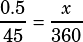The figure above shows part of a circle whose circumference is 45. If arcs of length 2 and length b continue to alternate around the entire circle so that there are 18 arcs of each length, what is the degree measure of each of the arcs of length b?

a) 4
b) 6
c) 10
d) 16
e) 20

You know that 18(2 + b) = 45, so you can calculate b:

18(2 + b) = 45
36 + 18b = 45
18b = 9
b = 0.5

Now all you need to do to find the degree measure of an arc of length b is set up a ratio, like you do for LOTS of circle problems.